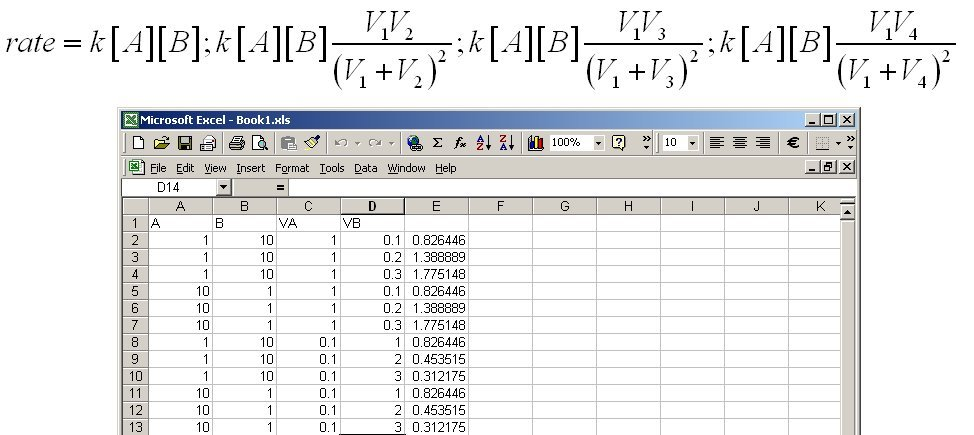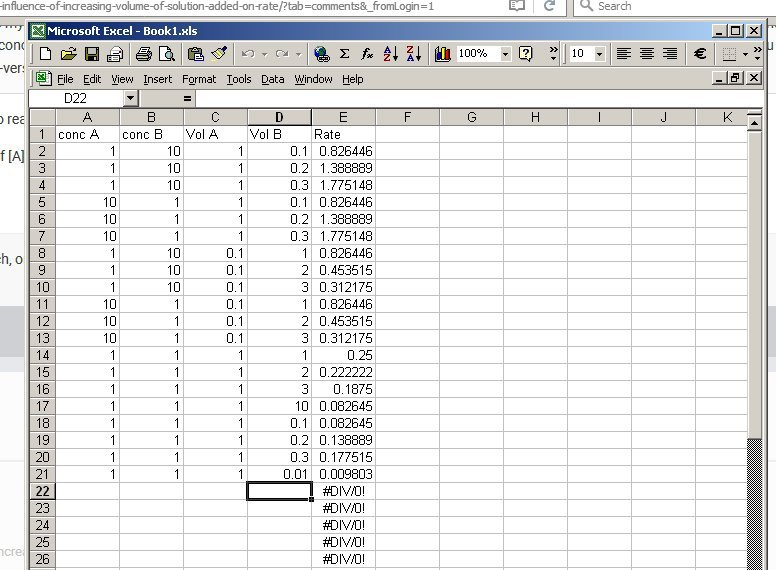## Recommended Posts

It's obvious why increasing the concentration of reactants increases rate.  What if you add simply more of a given reactant at a fixed concentration?  Should this also increase the rate?

Example: To 20ml of 0.1M A make three tests with 5ml, 10ml and 15ml of 1M B

Case 1: 2 x10-3 moles A and 5 x 10-3 moles B in 25ml = 0.28 moles reactants/L

Case 2: 2x10-3 moles A and 0,01moles B in 30 ml = 0.4 moles reactant/L

Case 3: 2x 10-3 moles A and 0.015moles B in 35ml = 0.485 moles reactant/L

So since we have more reactant moles/L as we add volume of reactant B at a fixed concentration the rate should increase, right?

Actually just thought, if you reversed the concentrations i.e. 20ml of 1M A and add 5ml, 10ml, 15ml of 0.1M B the rate should decrease as the reactants/L decrease when adding more volume.

So rate will either increase or decrease on adding more volume of a given reactant depending on relative concentrations, right?

##### Share on other sites
Posted (edited)
27 minutes ago, Bemused said:

It's obvious why increasing the concentration of reactants increases rate.

If you think so, perhaps you can say why ?

But you should also look at your definition of rate of reaction.

27 minutes ago, Bemused said:

What if you add simply more of a given reactant at a fixed concentration?  Should this also increase the rate?

Edited by studiot

##### Share on other sites
5 minutes ago, studiot said:

If you think so, perhaps you can say why ?

But you should also look at your definition of rate of reaction.

Well increasing concentration increases the number of reactant particles, increasing the number that have the required activation energy and increasing the frequency of collisions per unit volume.  The rate is a constant times the concentrations raised to powers dependent on the reaction mechanism so is therefore proportional to the concentration.

5 minutes ago, studiot said:

Could you elucidate why using the example I provided?  In the first case the concentration of reactants (moles/unit volume) increases which means the rate should increase.  When reversing the initial concentrations the rate decrease with increasing volume.

Thanks.

##### Share on other sites
2 minutes ago, Bemused said:

Well increasing concentration increases the number of reactant particles,

Not necessarily.

There are several considerations in play and you nearly have the theoretical justification leading to the following equation between reactants A and B.

Rate = Rate Constant x concentration of A x Concentration of B.

Theoretically we note that in order for a reaction between two molecules A and B to take place, they have to be in the same place at the same time.

So it is reasonable to suppose that the probability of a molecule being in a particular place at a particular time is proportional to the concentration (not the total number) of thaose molecules.

$rate \propto \left[ A \right]$

or

$rate = {c_A}\left[ A \right]$

But the rate is also proportional the probability of finding B in the same place is

$rate = {c_B}\left[ B \right]$

combining these we have

$rate = k\left[ A \right]\left[ B \right]$

Where k is the combined constant for both proportionalities.

But I also said look at the definition of 'rate'

Rate is the time rate of change of concentration (not total quantity) of one of the reactants

$rate = \frac{{d\left[ A \right]}}{{dt}} = \frac{{d\left[ B \right]}}{{dt}}$

This is why I said 'mathematically'.

But I also said theoretically.

In practice we find that experimentally this works for some reactions but for others the rate is actually proportional to some fractional power of the concentrations

$rate = k{\left[ A \right]^\alpha }{\left[ B \right]^\beta }$

Again sometimes we can deduce reasons for this but the ultimate arbiter is to measure alpha and beta.

##### Share on other sites

Ok so assuming the reaction rate is 1st order with respect to both reactants then the rate should vary according to how I have described it in the original post right?

##### Share on other sites
Posted (edited)
22 hours ago, Bemused said:

What if you add simply more of a given reactant at a fixed concentration?

If the concentration is fixed (ie constant) and the rate is proportional to the concentration how can the rate vary

IOW

$\frac{d}{{dt}}\left( {\frac{{d\left[ A \right]}}{{dt}}} \right) = 0$

Edited by studiot

##### Share on other sites

"If the concentration is fixed (ie constant) and the rate is proportional to the concentration how can the rate vary"

Maybe I'm not explaining myself well.  I am starting with fixed concentrations and then adding varying volumes of one to a fixed volume of the other.  Since the volumes are changing then the concentrations of the reactants must also be changing according to how I tried to describe it above.  Maybe I'm not framing the problem well or not following something in your answers to date.

##### Share on other sites
Posted (edited)
1 hour ago, Bemused said:

"If the concentration is fixed (ie constant) and the rate is proportional to the concentration how can the rate vary"

Maybe I'm not explaining myself well.  I am starting with fixed concentrations and then adding varying volumes of one to a fixed volume of the other.  Since the volumes are changing then the concentrations of the reactants must also be changing according to how I tried to describe it above.  Maybe I'm not framing the problem well or not following something in your answers to date.

I see.

So the answer now is it depends on the values.

So take the rate R = k[A]

and compare rate when you add V2 < V3 < V4 volumes of B to 3 samples of V1 volume of A.

Then the total volumes are now

V1 < (V1 + V2) < (V1 + V3) < (V1 + V4)

Which clearly dilutes [A] since you have added no more Ab ( that is V2, V3 and V4 contain no A)

So the initial concentrations for A to put into the rate equation are

$\left[ A \right] > \left[ A \right]\frac{{{V_1}}}{{\left( {{V_1} + {V_2}} \right)}} > \left[ A \right]\frac{{{V_1}}}{{\left( {{V_1} + {V_3}} \right)}} > \left[ A \right]\frac{{{V_1}}}{{\left( {{V_1} + {V_4}} \right)}}$

For B the situation works the other way round since each adds a large quantity of B to the total

So the initial quantities of B to put into the rate equation are

$\left[ B \right] > \left[ B \right]\frac{{{V_2}}}{{\left( {{V_1} + {V_2}} \right)}} > \left[ B \right]\frac{{{V_3}}}{{\left( {{V_1} + {V_3}} \right)}} > \left[ B \right]\frac{{{V_4}}}{{\left( {{V_1} + {V_4}} \right)}}$

So the rate varies depending and I will leave you to think about where it goes up or down.

But below is a spreadsheet tracking variations in [A], and the volumes of A and B.

$rate = k\left[ A \right]\left[ B \right];k\left[ A \right]\left[ B \right]\frac{{{V_1}{V_2}}}{{{{\left( {{V_1} + {V_2}} \right)}^2}}};k\left[ A \right]\left[ B \right]\frac{{{V_1}{V_3}}}{{{{\left( {{V_1} + {V_3}} \right)}^2}}};k\left[ A \right]\left[ B \right]\frac{{{V_1}{V_4}}}{{{{\left( {{V_1} + {V_4}} \right)}^2}}}$Edited by studiot

##### Share on other sites

Thanks for your efforts, so my initial post was essentially correct, the rate of reaction for two reacting species (assuming not zero order for either) will change according to adding different volumes of fixed concentration.  If the initial concentration of the reactant whose volume is held constant is larger then that of the reactant you add in increasing volume the rate will decrease and vice-versa.

##### Share on other sites
16 minutes ago, Bemused said:

Thanks for your efforts, so my initial post was essentially correct, the rate of reaction for two reacting species (assuming not zero order for either) will change according to adding different volumes of fixed concentration.  If the initial concentration of the reactant whose volume is held constant is larger then that of the reactant you add in increasing volume the rate will decrease and vice-versa.

I had though you might like to reason the condition out for yourself.

It depends both on the rato of [A] to and Vn to V1##### Share on other sites

Funnily enough I thought that was exactly what I did - what I wrote is analogous to the spreadsheet you (kindly) provided.

Much obliged.

## Create an account

Register a new account